# Binary Search Trees

The Binary Search Tree (BST) is a Binary Tree with the following properties.

1. Keys that are less than the parent are found in the left subtree
2. Keys that are greater than the parent are found in the right subtree
3. Both the left and right subtrees must also be binary search trees.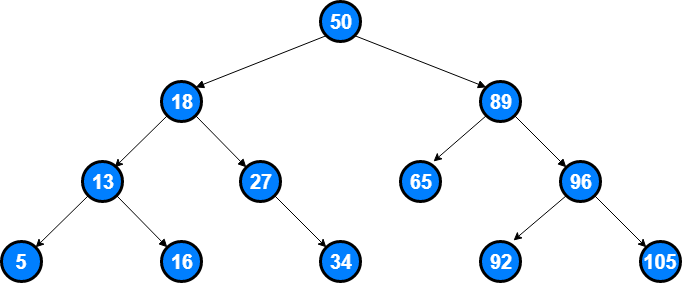## Binary Search Tree Operations

Operation Average Worst Case
Insert O(log n) O(n)
Lookup O(log n) O(n)
Delete O(log n) O(n)
Minimum O(log n) O(n)
Maximum O(log n) O(n)
Predecessor O(log n) O(n)
Successor O(log n) O(n)

When we are talking about the average case, it is the time it takes for the operation on a balanced tree, and when we are talking about the worst case, it is the time it takes for the given operation on a non-balanced tree.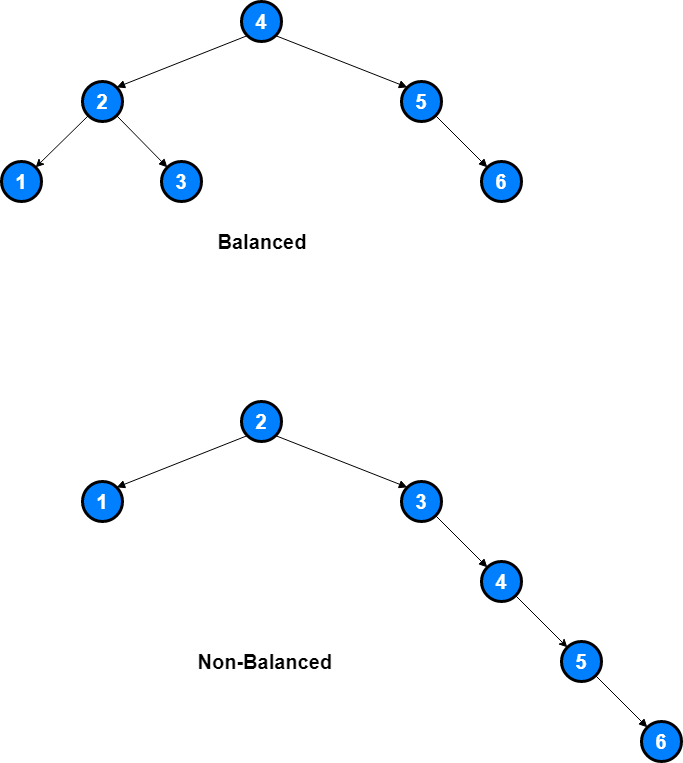## Implementation

We should start the implementation by defining `BinarySearchTree()` and `Node()` classes in our code.

An empty `BinarySearchTree()` class will look like this;

``````class BinarySearchTree:

def __init__(self):
self.root = None
self.size = 0

def length(self):
return self.size

def __len__(self):
return self.size
``````

and along with the `Node` class, we will implement lots of helper functions like; `has_left_child()`, `has_right_child()`, `is_left_child()`, `is_right_child()`, `is_root()`, `is_leaf()`, `has_any_children()`, `has_both_children()`, `splice_out()`, `find_successor()`, `find_min()`, `replace_node_data()` in order to make our future implementations more easier.

``````class Node:
def __init__(self, key, val, left=None, right=None, parent=None):
self.key = key
self.leftChild = left
self.rightChild = right
self.parent = parent

def has_left_child(self):
return self.leftChild

def has_right_child(self):
return self.rightChild

def is_left_child(self):
return self.parent and self.parent.leftChild == self

def is_right_child(self):
return self.parent and self.parent.rightChild == self

def is_root(self):
return not self.parent

def is_leaf(self):
return not (self.rightChild or self.leftChild)

def has_any_children(self):
return self.rightChild or self.leftChild

def has_both_children(self):
return self.rightChild and self.leftChild
``````

### Insert Operation

Since we now have a `Node()` and `BinarySearchTree()` classes, we are ready to insert elements to this `BinarySearchTree()` class.

We are going to implement a `put(self, key, val)` method. This method will check to see if the tree already has a root. If there is not a root then `put()` will create a new `Node()` and install it as the root of the tree. If a root node is already in place then `put()` calls the private, recursive, helper function `_put()` to search the tree according to the Binary Search Tree properties that we explained in the first paragraph of this article.

``````def put(self, key, val):
if self.root:
self._put(key, val, self.root)
else:
self.root = Node(key, val)
self.size = self.size + 1

def _put(self, key, val, current_node):
if key < current_node.key:
if current_node.has_left_child():
self._put(key, val, current_node.leftChild)
else:
current_node.leftChild = Node(key, val, parent=current_node)
else:
if current_node.has_right_child():
self._put(key, val, current_node.rightChild)
else:
current_node.rightChild = Node(key, val, parent=current_node)

def __setitem__(self, k, v):
self.put(k, v)
``````

### Lookup (Search) Operation

Once the tree is constructed, the next task is to implement the retrieval of a value for a given key. The `get()` method is even easier than the `put()` method because it simply searches the tree recursively until it gets to a non-matching leaf node or finds a matching key. When a matching key is found, the value stored in the payload of the node is returned.

``````def get(self, key):
if self.root:
result = self._get(key, self.root)
if result:
else:
return None
else:
return None

def _get(self, key, current_node):
if not current_node:
return None
elif current_node.key == key:
return current_node
elif key < current_node.key:
return self._get(key, current_node.leftChild)
else:
return self._get(key, current_node.rightChild)

def __getitem__(self, key):
return self.get(key)

def __contains__(self, key):
if self._get(key, self.root):
return True
else:
return False
``````

### Delete Operation

`delete()` operation is the most challenging operation in the Binary Search Tree.

The first task is to find the node to delete by searching the tree. If the tree has more than one node we search using the `_get()` method to find the `Node()` that needs to be removed. If the tree only has a single node, that means we are removing the root of the tree, but we still must check to make sure the key of the root matches the key that is to be deleted.

In either case if the key is not found the `delete()` method raises an error.

``````def delete(self, key):
if self.size > 1:
node_to_remove = self._get(key, self.root)
if node_to_remove:
self.remove(node_to_remove)
self.size = self.size - 1
else:
raise KeyError('Error, key not in tree')
elif self.size == 1 and self.root.key == key:
self.root = None
self.size = self.size - 1
else:
raise KeyError('Error, key not in tree')

def __delitem__(self, key):
self.delete(key)
``````

Once we have found the node containing the key we want to delete, there are three cases that we must consider:

1. The node to be deleted has no children
2. The node to be deleted has only one child
3. The node to be deleted has two children

Handling the first case is pretty easy: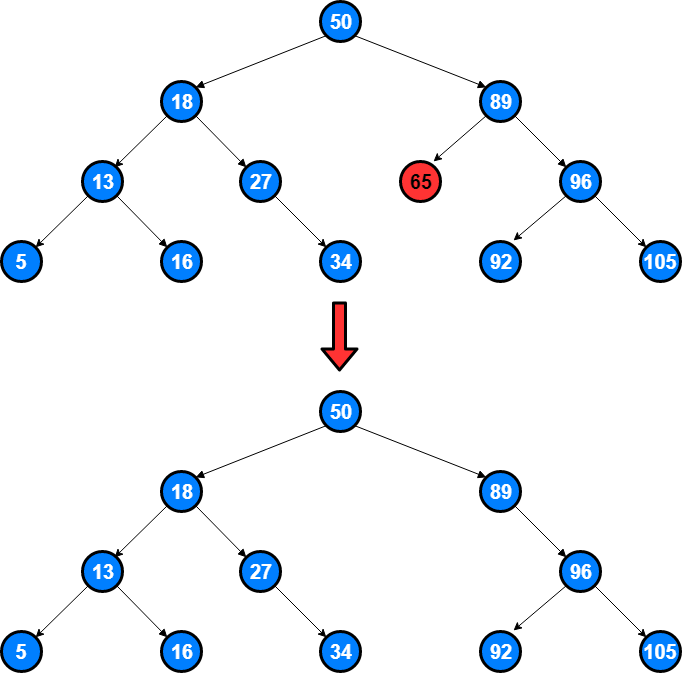``````def remove(self, current_node):
if current_node.is_leaf():  # leaf
if current_node == current_node.parent.leftChild:
current_node.parent.leftChild = None
else:
current_node.parent.rightChild = None
``````

If a node has only a single child, then we can simply promote the child to take the place of its parent.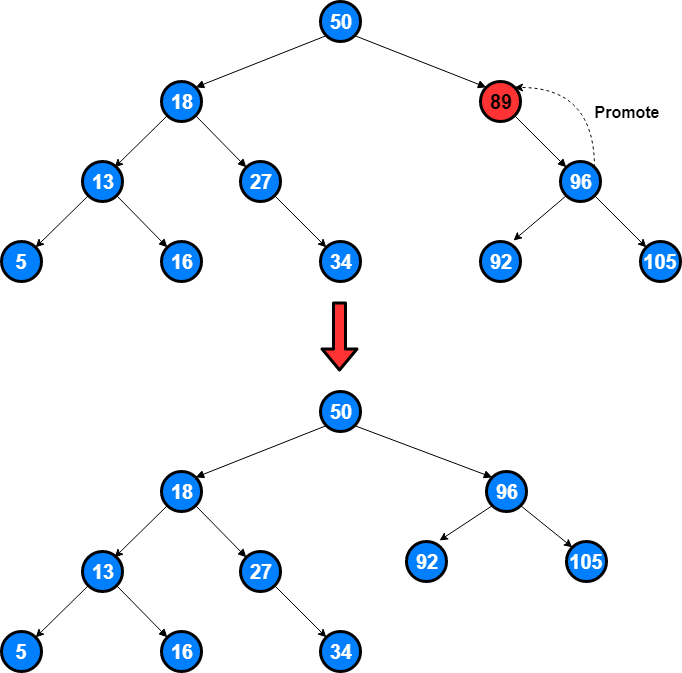The decision proceeds as follows:

1. If the current node is a left child then we only need to update the parent reference of the left child to point to the parent of the current node, and then update the left child reference of the parent to point to the current node’s left child.
2. If the current node is a right child then we only need to update the parent reference of the left child to point to the parent of the current node, and then update the right child reference of the parent to point to the current node’s left child.
3. If the current node has no parent, it must be the root. In this case we will just replace the `key`, `payload`, `leftChild`, and `rightChild` data by calling the `replace_node_data()` method on the `root`.
``````else:  # this node has one child
if current_node.has_left_child():
if current_node.is_left_child():
current_node.leftChild.parent = current_node.parent
current_node.parent.leftChild = current_node.leftChild
elif current_node.is_right_child():
current_node.leftChild.parent = current_node.parent
current_node.parent.rightChild = current_node.leftChild
else:
current_node.replace_node_data(current_node.leftChild.key,
current_node.leftChild.leftChild,
current_node.leftChild.rightChild)
else:
if current_node.is_left_child():
current_node.rightChild.parent = current_node.parent
current_node.parent.leftChild = current_node.rightChild
elif current_node.is_right_child():
current_node.rightChild.parent = current_node.parent
current_node.parent.rightChild = current_node.rightChild
else:
current_node.replace_node_data(current_node.rightChild.key,
current_node.rightChild.leftChild,
current_node.rightChild.rightChild)
``````

If a node has two children, then it is unlikely that we can simply promote one of them to take the node’s place. We can, however, search the tree for a node that can be used to replace the one scheduled for deletion.

What we need is a node that will preserve the binary search tree relationships for both of the existing left and right subtrees. The node that will do this is the node that has the next-largest key in the tree. We call this node the successor, and we will look at a way to find the successor shortly.

The successor is guaranteed to have no more than one child, so we know how to remove it using the two cases for deletion that we have already implemented.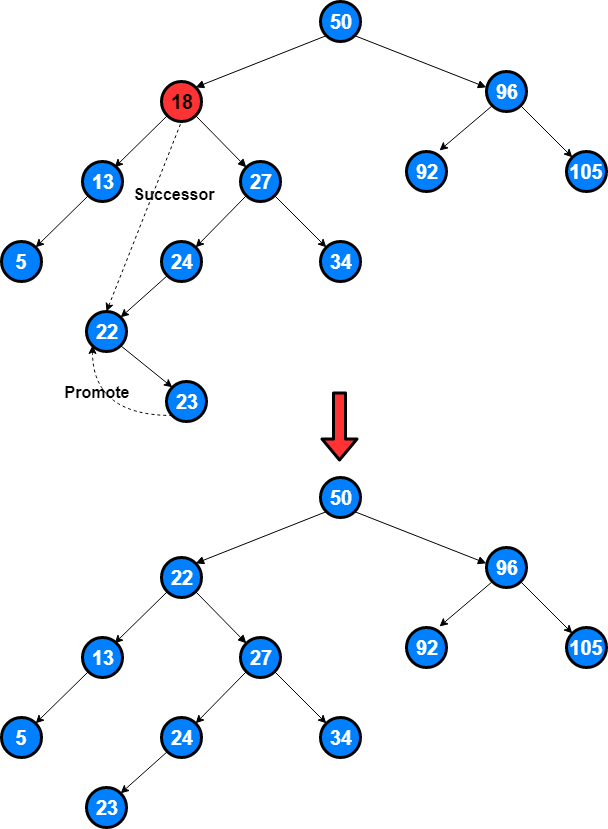``````elif current_node.has_both_children():
successor = current_node.find_successor()
successor.splice_out()
current_node.key = successor.key
``````

There are three cases to consider when looking for the successor:

1. If the node has a right child, then the successor is the smallest key in the right subtree
2. If the node has no right child and is the left child of its parent, then the parent is the successor
3. If the node is the right child of its parent, and itself has no right child, then the successor to this node is the successor of its parent, excluding this node.
``````def splice_out(self):
if self.is_leaf():
if self.is_left_child():
self.parent.leftChild = None
else:
self.parent.rightChild = None
elif self.has_any_children():
if self.has_left_child():
if self.is_left_child():
self.parent.leftChild = self.leftChild
else:
self.parent.rightChild = self.leftChild
self.leftChild.parent = self.parent
else:
if self.is_left_child():
self.parent.leftChild = self.rightChild
else:
self.parent.rightChild = self.rightChild
self.rightChild.parent = self.parent

def find_successor(self):
successor = None
if self.has_right_child():
successor = self.rightChild.find_min()
else:
if self.parent:
if self.is_left_child():
successor = self.parent
else:
self.parent.rightChild = None
successor = self.parent.find_successor()
self.parent.rightChild = self
return successor

def find_min(self):
current = self
while current.has_left_child():
current = current.leftChild
return current

def replace_node_data(self, key, value, lc, rc):
self.key = key
self.leftChild = lc
self.rightChild = rc
if self.has_left_child():
self.leftChild.parent = self
if self.has_right_child():
self.rightChild.parent = self
``````

## Full Implementation Code

Full implementation code can be found at my GitHub page.

Tags:

Categories:

Updated: